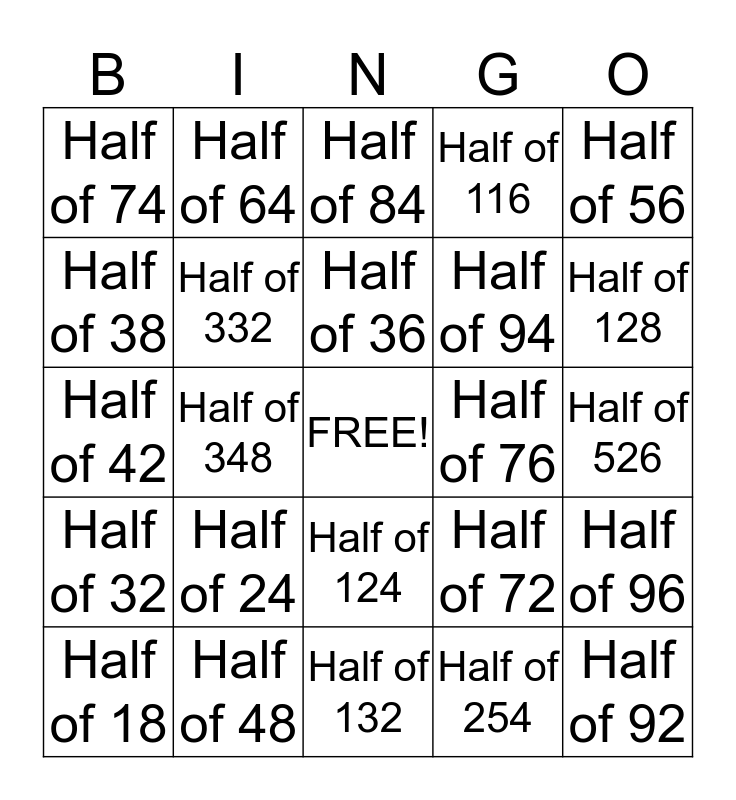# Halving BingoThis bingo card has a free space and 24 words: Half of 24, Half of 48, Half of 36, Half of 18, Half of 56, Half of 74, Half of 38, Half of 64, Half of 76, Half of 124, Half of 94, Half of 42, Half of 72, Half of 96, Half of 332, Half of 254, Half of 526, Half of 84, Half of 128, Half of 348, Half of 92, Half of 32, Half of 116 and Half of 132.

## Play Online